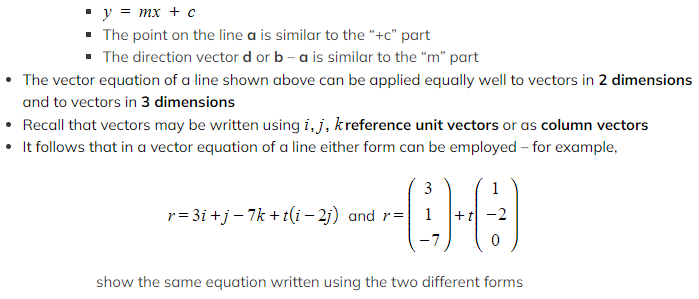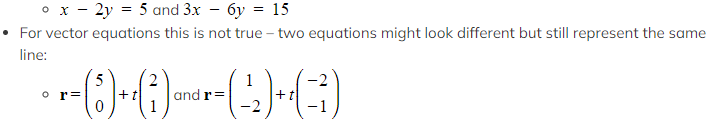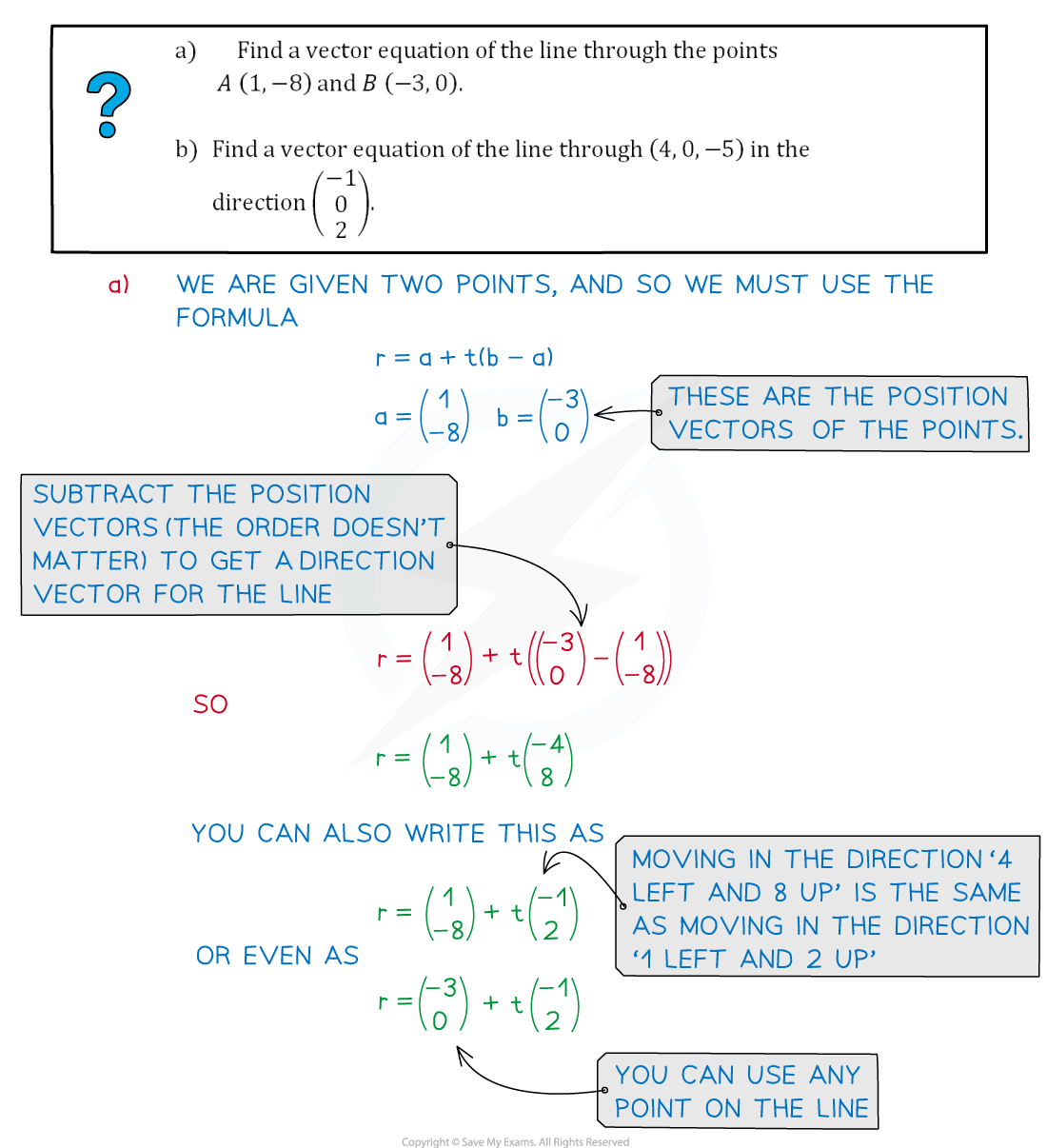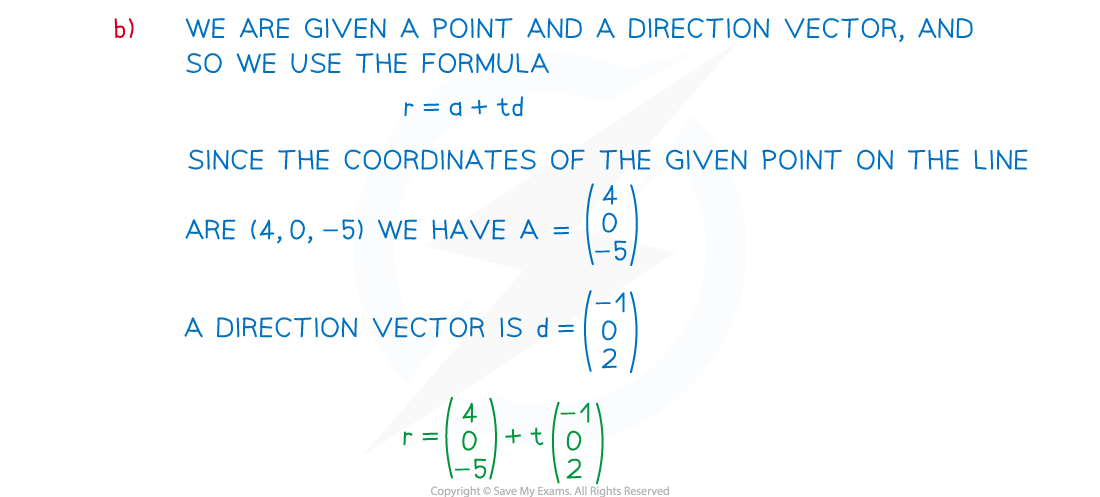# CIE A Level Maths: Pure 3复习笔记7.3.1 Equation of a Line in Vector Form

### Equation of a Line in Vector Form

#### How do I find the vector equation of a line?

• You need to know:
• The position vector of one point on the line
• direction vector of the line (or the position vector of another point)
• There are two formulas for getting a vector equation of a line:
• a + t (b - a)
• use this formula when you know the position vectors a and b of two points on the line
• a + t d
• use this formula when you know the position vector a of a point on the line and a direction vector d
• Both forms could be compared to the Cartesian equation of a 2D line

####How do I determine if a point is on a line?

• Each different point on the line corresponds to a different value of t
• For example: if an equation for a line is r = 3i + 2j - + t (i + 2j)
• the point with coordinates (2, 0, -1) is on the line and corresponds to t = -1
• However we know that the point with coordinates (-7, 5, 0) is not on this line
• No value of could make the k component 0

#### Can two different equations represent the same line?

• Why do we say direction vector and not the direction vector? Because the magnitude of the vector doesn’t matter; only the direction is important
• we can multiply any direction vector by a (non-zero) constant and this wouldn’t change the direction
• Therefore there are an infinite number of options for (a point on the line) and an infinite number of options for the direction vector
• For Cartesian equations – two equations will represent the same line only if they are multiples of each other

####Worked Example#### Exam Tip

• Remember that the vector equation of a line can take many different forms. This means that the answer you derive might look different from the answer in a mark scheme.
• You can choose whether to write your vector equations of lines using reference unit vectors or as column vectors – use the form that you prefer!
• If, for example, an exam question uses column vectors, then it is usual to leave the answer in column vectors, but it isn’t essential to do so - you’ll still get the marks!